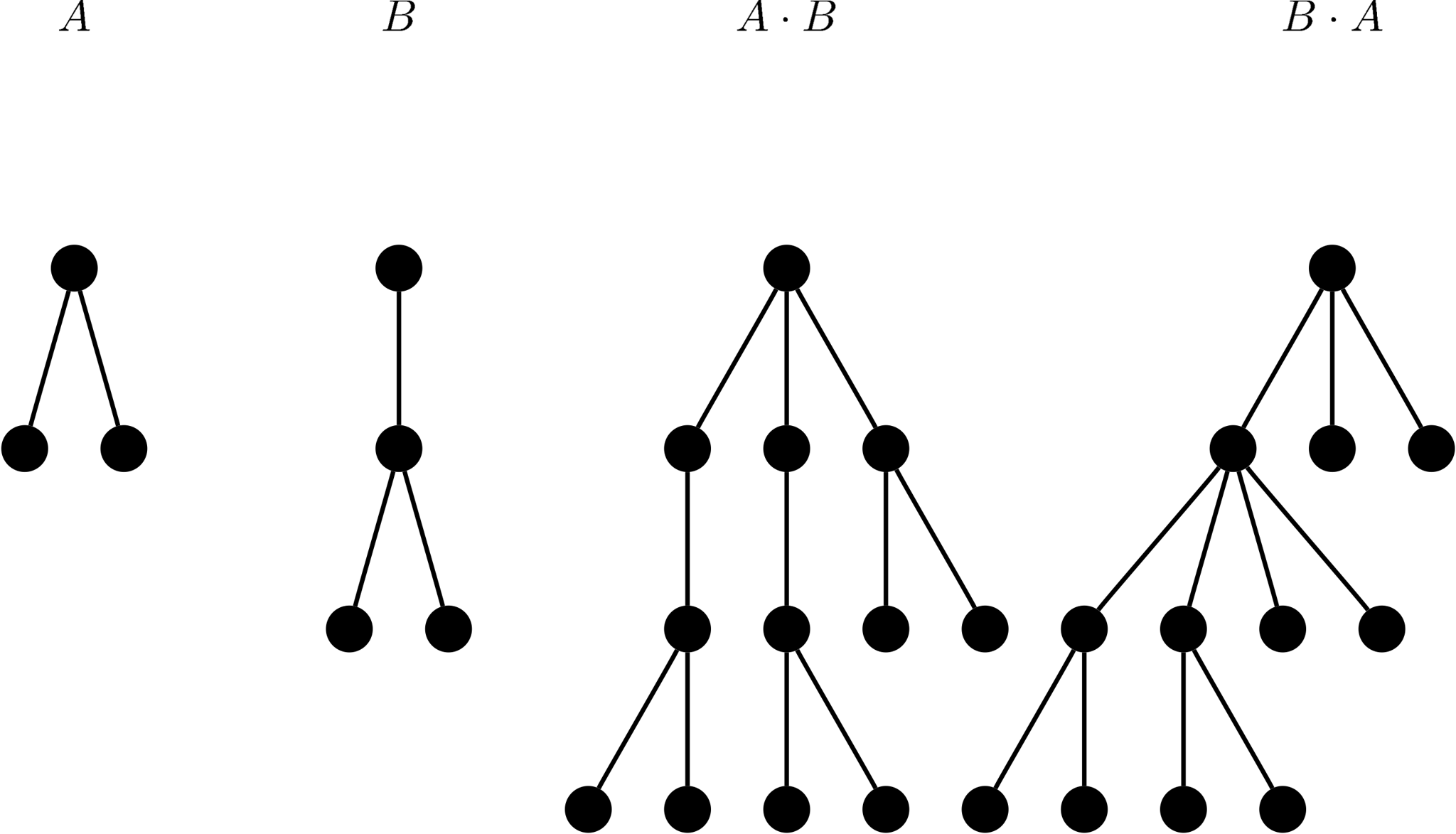시간 제한메모리 제한제출정답맞힌 사람정답 비율
2 초 512 MB7000.000%

문제

Bobo proposes a multiplication operation on rooted trees.

Let $A$ and $B$ be two arbitrary rooted trees. Then $T = A \cdot B$ is built by making a copy of $B$ for each vertex $x \in A$ and merging the root of this copy with $x$ (see the following figure for more details). We then call $A$ and $B$ factors of $T$.Apparently, we have $T \cdot \mathbf{1} = \mathbf{1} \cdot T = T$, where $\mathbf{1}$ is the rooted tree with only one vertex. So, $\mathbf{1}$ is a factor of every rooted tree, and every rooted tree is a factor of itself. And if a rooted tree $T$ only has $T$ and $\mathbf{1}$ as his factors, we call $T$ a prime tree.

Bobo has a rooted tree $T$ with $n$ nodes which are conveniently labeled with $1, 2, \dots, n$. He wants to factor $T$ into multiplication of as many prime trees as possible (that is, find an equation $T = T_1 \cdot T_2 \cdots T_m$ where $T_i$ ($1 \leq i \leq m$) are prime trees and $m$ is maximum).

Note that $\mathbf{1}$ is not a prime tree.

입력

The input contains zero or more test cases, and is terminated by end-of-file. For each test case:

The first line contains an integer $n$, the number of nodes ($2 \leq n \leq 10^6$).

The second line contains $(n - 1)$ integers $p_2, p_3, \dots, p_n$, where $p_i$ is the parent of the $i$-th node ($1 \leq p_i \leq i - 1$).

It is guaranteed that the sum of all $n$ does not exceed $10^6$.

출력

For each test case, output an integer denoting the maximum number of prime factors.

예제 입력 1

12
1 1 1 1 2 2 4 5 5 6 10
3
1 1
6
1 1 1 2 3
13
1 1 1 2 2 3 3 4 5 6 7 8

3
1
2
1